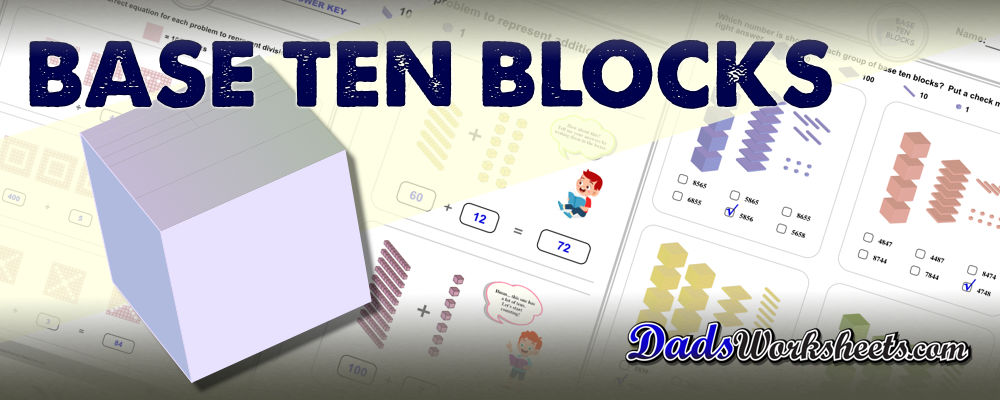# Teaching Math with Base Ten Blocks

Base ten blocks are a way for visual learners to grasp quantities, and while they frequently take the form of physical manipulatives in the classroom, these ew printable base ten block worksheets can inspire the same understanding.

Base 10 blocks are visual representations of numbers that help students learn the concepts of quantity and place value. The fundamental unit of a system of base ten blocks is the unit cube, which is a stand in for a quantity of one. A stack of ten units into a rod represents a value in the tens place. Similarly, ten rods form a flat that represents the quantity one hundred, and a larger cube constructed from ten flats represents one thousand.

A student seeing these units, rods, flats and cubes together can easily understand how a number is composed, and counting up each base 10 primitive gives the plave value for a number written out in regular Arabic decimal form. The different base ten block shapes are often represented using different colors to emphasize their different place value roles in composing base 10 numbers.

In the spirit of a picture being worth 1000, words, check the link below to see what I mean...

#### Base Ten Blocks

You can check out all of these new worksheets at the link above... Be sure to take a look at the place value chart, place value worksheets, printable number line and printable hundreds chart pages for other tools to teach place value and number sense!